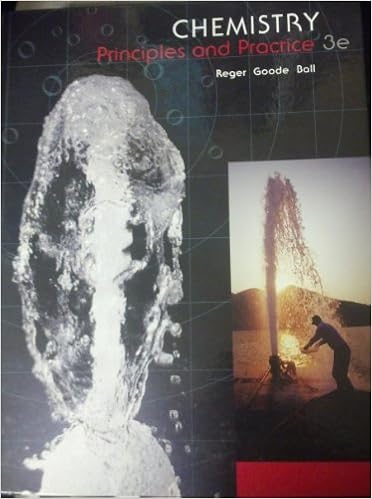# A solid produced by a chemical reaction in solution

• Notes
• 22
• 50% (2) 1 out of 2 people found this document helpful

This preview shows page 5 - 8 out of 22 pages.

##### We have textbook solutions for you!
The document you are viewing contains questions related to this textbook.The document you are viewing contains questions related to this textbook.
Chapter 3 / Exercise 3.64
Chemistry: Principles and Practice
Reger ChemExpert Verified
88. A solid produced by a chemical reaction in solution that separates from the solution is called a. a precipitate. c. a molecule. b. a reactant. d. the mass of the product.
##### We have textbook solutions for you!
The document you are viewing contains questions related to this textbook.The document you are viewing contains questions related to this textbook.
Chapter 3 / Exercise 3.64
Chemistry: Principles and Practice
Reger ChemExpert Verified
____ 89. After the correct formula for a reactant in an equation has been written, the
____ 90. What is the small whole number that appears in front of a formula in a chemical equation?
____ 91. According to the law of conservation of mass, the total mass of the reacting substances is
____ 92. A chemical equation is balanced when the a. coefficients of the reactants equal the coefficients of the products. b. same number of each kind of atom appears in the reactants and in the products. c. products and reactants are the same chemicals. d. subscripts of the reactants equal the subscripts of the products.
____ 93. Which word equation represents the reaction that produces water from hydrogen and oxygen?
____ 94. Which of the following is a formula equation for the formation of carbon dioxide from carbon and oxygen?
2
2 ____ 95. In an equation, the symbol for a substance in water solution is followed by
).
____ 96. Which coefficients correctly balance the formula equation NH4NO2(s)N2(g) + H2O(l)? a. 1, 2, 2 c. 2, 1, 1 b. 1, 1, 2 d. 2, 2, 2
____ 97. Which coefficients correctly balance the formula equation CaO + H2O Ca(OH)2?
____ 98. After the first steps in writing an equation, the equation is balanced by
____ 99. Which equation is NOT balanced?
2O
O
•••